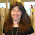## Monday, December 22, 2014

### Homomorphicly Encrypted Bitcoin Outputs for Transaction Anonymity

#### There has been some loosely organized comments regarding the possibility of using Homomorphic Encryption to achieve a new sort of anonymity for Bitcoin.

Is this possible?  I have a hunch it is possible to use some HE techniques to achieve a new level of anonymity for Bitcoin.  But first: what is Homomorphic Encryption?

### Homomorphic Encryption

HE is a sort of encryption that allows for operations on data(such as arithmetic) while the data remains encrypted.

ex.  we encrypt two integers X and Y yielding X' and Y'.

X' and Y' are unknowable to anyone but the possessor of a private key.

we can perform an operation X' + Y' yielding Z'.  Z' is unknowable to the entity which performs this operation and that is the key use case.

### How Can This Be Used For Bitcoin?

Presumably, we can encrypt the values of bitcoin outputs, making the actual cash flows invisible to the public.  It would be in essence similar to a cash economy where all transactions are conducted using sealed envelopes.  The public would we aware that a transaction took place, but the amount would be invisible.  What is required though is a special sort of Homomorphic Encryption

1) simple arithmetic must be possible on the HE'd outputs (this is at this time strongly supported in theory)

2) the HE'd outputs must be visible to the recipient ie. decryptable with their public key

### How Would This Technique Achieve Anonymity?

Using this basic technique it would be impossible for anyone to tell what the balances of accounts may be at any point in the blockchain.  It would be fairly easy to confuse auditors and passive surveillance operations by making many small transactions to feint attempts at detecting fund transfers.

### Is this different than Mixers?

Technologies such as Zerocoin achieve similar but qualitatively different results.  These techniques may be used in conjunction with Homomorphicly Encrypted Outputs.

#### 1 comment:

1.Homomorphic encryption is really, really slow. (I was excited about it when I read the "inventor" guy, Craig Gentry's description of it, in 2013.) It wouldn't be useful for financial transactions processing, not yet, I don't think.

```##################################
##################################
##################################
##################################
##################################
##################################
##################################
##################################
##################################
##################################
##################################
##################################
##################################
##################################
##################################
##################################
##################################
##################################
##################################
##################################
##################################
##################################
##################################
##################################
##################################
##################################
##################################
##################################
```